# D分离——贝叶斯网络

## 基本概念

D分离的概念来自于贝叶斯网络,是用来寻找条件独立的有效方法

$$P(A|B,C)=P(A|B)$$

## 有向网络连接中节点间的三个基本关系

### (1) 串行连接

​ 在串行连接中A通过事件B影响C，同样事件C也是用过事件B影响A。我们认为当证据B确定时，A、C条件独立。### (2) 分叉连接

​ 在分叉连接中A影响子节点，同样子节点通过A影响其他子节点。我们认为当A已知时，其各个子节点相互独立。称B、C、...、Z被A节点D分离。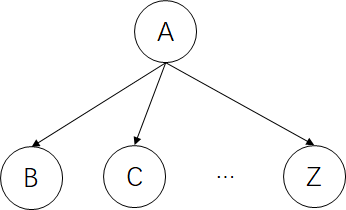### (3) 汇集连接（比较特殊）

​ 在汇集连接中只有A节点未知时，我们才能认为其父节点们相互独立。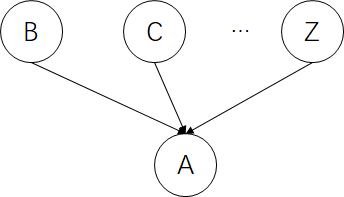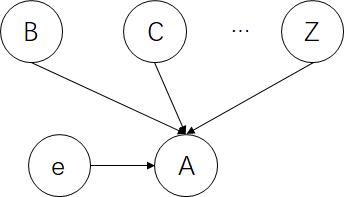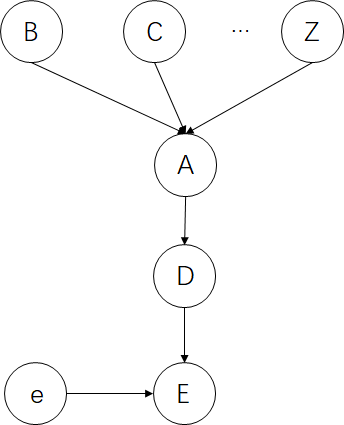## D分离的条件

1. $$V_{b}$$在𝛆中，且路径上的两条弧都以$$V_{b}$$结尾。 （$$V_{b}$$相当于分叉连接中的A节点）
2. $$V_{b}$$在𝛆中，且路径上的一条弧以$$V_{b}$$为头，一条弧以$$V_{b}$$结尾。 （$$V_{b}$$相当于串行连接中的B节点）
3. $$V_{b}$$及其后代结点都不在𝛆中，且路径上的两条弧都以$$V_{b}$$为头。（$$V_{b}$$相当于汇集连接中的A节点）

## 实例分析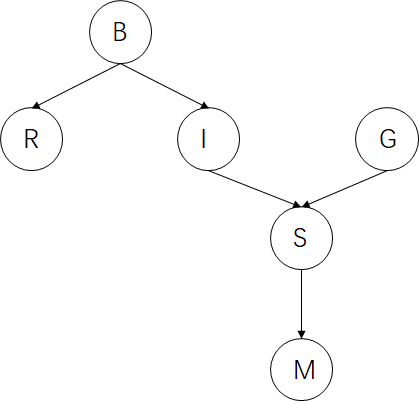$$P(B,R,I,G,S,M)=P(M|B,R,I,G,S)*P(S|B,R,I,G)*P(G|B,R,I)*P(I|B,R)*P(R|B)*P(B)$$

$$P(B,R,I,G,S,M)=P(M|S)*P(S|I,G)*P(G)*P(I|B)*P(R|B)*P(B)$$

$$P(M|B,R,I,G,S)$$，可以被这样解决，给定节点B,R,I,G,S（可视为证据结点），判断它与那些节点条件独立，然后按照条件独立性化简。

• M,S直接连接，必然有影响，不独立。
• 给定S后，B,R,I,G.到M的所有无向路径被S阻塞。按照条件独立性$$P(M|B,R,I,G,S)=P(M|S)$$

$$P(S|B,R,I,G)$$，同理。

• I,G和S直连，必然相互影响，不独立。
• 给定I后，B,R到S的所有无向路径被I阻塞。B,R和I条件独立，按照条件独立性$$P(S|B,R,I,G)=P(S|I,G)$$

$$P(G|B,R,I)$$,同理

• B,R,I中没有结点阻塞G到B,R,I的所有无向路径。因为S没有给出且S的后代结点没有被给出结点B,R,I影响。所以B,R,I和G条件独立。$$P(G|B,R,I)=P(G)$$

$$P(I|B,R)$$,同理

• 给定B后，RBI路径被B阻塞，所以R，I在B的条件下独立。$$P(I|B,R)=P(I|B)$$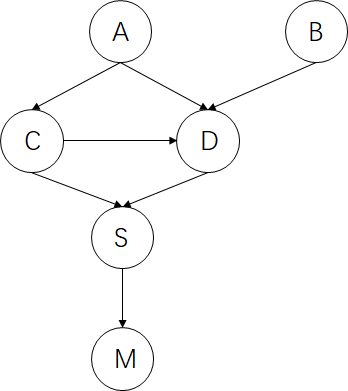a) 列出从B到S所有无向路径

b) 给定证据结点D,那么a)中哪些无向路径被阻塞？

c) 等式$$P(S|B,D)=P(S|D)$$是否成立，为什么？

a) 从B到S利用深度优先搜索的思路找到所有无向路径。

​ BDS,BDCS,BDACS

b) BDS,被D阻塞（B,S串行连接被D阻塞）；BDACS,未被阻塞(A,B汇集连接处D已知，路径未阻塞)；BDCS,未被阻塞(C,B汇集连接处D已知，路径未阻塞)；

c) 由b)可知，B,S之间所有无向路径并未都被阻塞，B,S并不条件独立，所以等式$$P(S|B,D)=P(S|D)$$不成立。

posted @ 2020-01-13 11:54  SheepHuan  阅读(3741)  评论(1编辑  收藏  举报